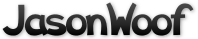Got questions, comments, patches, etc.? Contact Jason Woofenden
 author Jason Woofenden Mon, 25 Nov 2013 21:07:47 +0000 (16:07 -0500) committer Jason Woofenden Mon, 25 Nov 2013 21:07:47 +0000 (16:07 -0500)
 main.coffee patch | blob | history

index 860dd5d..14c8cb5 100644 (file)
@@ -370,6 +370,106 @@ unsink = (tile) ->
tile.text = new_letter().letter
tile.dom.html tile.text

+
+default_booms = {
+       3: {
+               neighbors: {
+                       flips: 1
+                       force: 2
+               }
+               neighbor_neighbors: {
+                       flips: 0
+                       force: 0
+               }
+               board: {
+                       flips: 0
+                       force: 0
+               }
+       }
+       4: {
+               neighbors: {
+                       flips: 'all'
+                       force: 4
+               }
+               neighbor_neighbors: {
+                       flips: 0
+                       force: 2
+               }
+               board: {
+                       flips: 0
+                       force: 0
+               }
+       }
+       5: {
+               neighbors: {
+                       flips: 'all'
+                       force: 6
+               }
+               neighbor_neighbors: {
+                       flips: 2
+                       force: 4
+               }
+               board: {
+                       flips: 0
+                       force: 0
+               }
+       }
+       6: {
+               neighbors: {
+                       flips: 'all'
+                       force: 10
+               }
+               neighbor_neighbors: {
+                       flips: 5
+                       force: 6
+               }
+               board: {
+                       flips: 0
+                       force: 0
+               }
+       }
+       7: {
+               neighbors: {
+                       flips: 'all'
+                       force: 10
+               }
+               neighbor_neighbors: {
+                       flips: 'all'
+                       force: 10
+               }
+               board: {
+                       flips: 0
+                       force: 2
+               }
+       }
+       lots: {
+               neighbors: {
+                       flips: 0
+                       force: 0
+               }
+               neighbor_neighbors: {
+                       flips: 0
+                       force: 0
+               }
+               board: {
+                       flips: 'all'
+                       force: 10
+               }
+       }
+}
+booms = null
+init_booms = ->
+       booms = {}
+       for  level, areas of default_booms
+               new_level = {}
+               for area, effects of areas
+                       new_area = {}
+                       for effect, amount of effects
+                               new_area[effect] = amount
+                       new_level[area] = new_area
+               booms[level] = new_level
+       return
+
# remove the selected tiles from the board, create new tiles, and slide everything into place
blip_selection = ->
word_length = selected_word().length
@@ -394,77 +494,51 @@ blip_selection = ->
# convert to arrays so we can sort, etc
nneighbors = (v for k, v of nneighbors)
neighbors = (v for k, v of neighbors)
-       boom = [
-               {
-                       tiles: neighbors,
-                       up: [],
+       areas = {
+               neighbors: {
+                       tiles: neighbors
+                       up: []
+                       down: []
+               }
+               neighbor_neighbors: {
+                       tiles: nneighbors
+                       up: []
down: []
-               },
-               {
-                       tiles: nneighbors,
-                       up: [],
+               }
+               board: {
+                       tiles: (space.tile for space in spaces)
+                       up: []
down: []
}
-       ]
-       for n in boom
-               for t in n.tiles
+       }
+       for k, v of areas
+               for t in v.tiles
if t.hp is 0
-                               n.down.push t
+                               v.down.push t
else
-                               n.up.push t
-       switch word_length
-               when 3
-                       boom.flips = 1
-                       boom.force = 2
-                       boom.flips = 0
-                       boom.force = 0
-               when 4
-                       boom.flips = 'all'
-                       boom.force = 4
-                       boom.flips = 0
-                       boom.force = 2
-               when 5
-                       boom.flips = 'all'
-                       boom.force = 6
-                       boom.flips = 2
-                       boom.force = 4
-               when 6
-                       boom.flips = 'all'
-                       boom.force = 10
-                       boom.flips = 5
-                       boom.force = 6
-               when 7
-                       boom.flips = 'all'
-                       boom.force = 10
-                       boom.flips = 'all'
-                       boom.force = 10
-               else
-                       boom.flips = 0
-                       boom.force = 0
-                       boom.flips = 0
-                       boom.force = 0
-                       # unsink/heal the whole board
-                       for s in spaces
-                               if s.tile.hp is 0
-                                       unsink s.tile
-                               else
-                                       s.tile.new_hp = 10
-       for b in boom
-               if b.flips is 'all' or b.flips >= b.down.length
-                       for t in b.down
+                               v.up.push t
+       if word_length < 8
+               boom = booms[word_length]
+       else
+               boom = booms.lots
+       for area_name, effects of boom
+               area = areas[area_name]
+               if effects.flips is 'all' or effects.flips >= area.down.length
+                       for t in area.down
unsink t
else
-                       down_count = b.down.length
-                       while b.flips > 0 and down_count
-                               b.flips -= 1
+                       down_count = area.down.length
+                       flips_left = effects.flips
+                       while flips_left > 0 and down_count > 0
+                               flips_left -= 1
flipper = Math.floor(Math.random() * down_count)
-                               unsink b.down[flipper]
+                               unsink area.down[flipper]
down_count -= 1
# move the last tile back into range
-                               b.down[flipper] = b.down[down_count]
-               if b.force > 0
-                       for t in b.up
-                               t.new_hp = t.hp + b.force
+                               area.down[flipper] = area.down[down_count]
+               if effects.force > 0
+                       for tile in area.up
+                               tile.new_hp = tile.hp + effects.force
for s in spaces
s.tile.new_hp ?= s.tile.hp - 1
if s.tile.new_hp < 0
@@ -721,6 +795,7 @@ start_over = ->
for s in spaces
selected.push s.tile
blip_selection()
+       init_booms()

init_start_over_link = ->
\$('#start-over').click (event) ->
@@ -761,5 +836,6 @@ init_game = ->
init_html_board()
init_start_over_link()
update_selection_display()
+       init_booms()

\$(init_game)